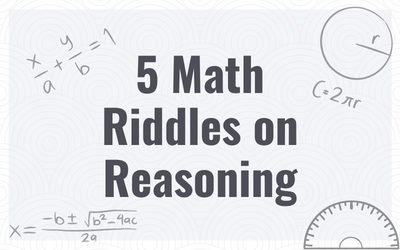# Here Are 5 Math Riddles On Reasoning To Prepare You For Your ExamsHere Are 5 Math Riddles On Reasoning To Prepare You For Your Exams

Math riddles are fun and challenging. Solving them helps develop logical thinking skills and improves memory. They also improve concentration and focus.

This is why we are here with a set of math riddles based on reasoning to help you prepare for any exam, academic or competitive.

Let’s begin.

## Here Are 5 Math Riddles On Reasoning To Prepare You For Your Exams

Math Riddle #1

Math Riddle #2

Math Riddle #3

Math Riddle #4

Math Riddle #5

Math Riddle #1

In the 1st circle, (10 - 3) x 5 = 35

In the 2nd circle, (6 - 4) x 8 = 16

Similarly, in the 3rd circle, (3 - 2) x 12 = 12

Math Riddle #2

2 - 2 x 3 +3

=> 2 - 6 + 3

=> 2 - 3

=> -1

Math Riddle #3

In the 1st column, 20 + 48 + 14 = 82

In the 3rd column, 39 + 20 + 25 = 82

Similarly in the 3rd column, 13 + 22 + ? = 82

Hence, ? = 47

Math Riddle #4

3 x 1 = 3

3  x 2 = 6

6 x 3 = 18

18 x 4 = 72

72 x 5 = 360

Math Riddle #5

Add the number of letters in each digit and you’ll get the sum on the R.H.S.

Hence, 7 + 9 = FIVE + FOUR = 9

We hope you liked this math riddle. Also Try:

Try And Solve This Math Riddle That Has Stumped Even Engineers.

Math Riddle: Even Math Teachers Have Failed To Solve This Viral Stolen Bill Problem. Can You?

Bored Of Tough Problems? Here Are Fun Math Riddles For Adults.

Math Riddle: Can You Solve This Children's Math Problem That Has Puzzled Even Adults?